# McKay-Alperin conjecture

Letbe a finite group. For any prime number, letbe the number of irreducible complex characters ofwith degree prime to(cf. also Character of a group). The simplest form of the McKay–Alperin conjectures asserts that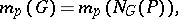whereis a Sylow-subgroup ofand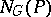is its normalizer (cf. also Sylow subgroup;-group; Normalizer of a subset). J. McKay [a2] first suggested this might be true whenis a simple group. J.L. Alperin [a1] observed that it is probably true for all finite groups.

Alperin also made a more general conjecture, involving characters in-blocks. (See Brauer first main theorem for notation and definitions.) Letbe a-block ofwith defect group(cf. Defect group of a block), and letbe an irreducible character (cf. also Irreducible representation) belonging to. Letbe a Sylow-subgroup of. By a theorem of R. Brauer,divides the degree. The characteris said to have height zero if the largest power ofdividing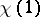is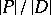. The more general Alperin conjecture asserts that the number of irreducible characters of height zero inis equal to the number of irreducible characters of height zero in the unique block of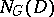sent toby the Brauer correspondence.

The conjectures are still not proved (1998), but the evidence in their favour is very strong.

How to Cite This Entry:
McKay-Alperin conjecture. Encyclopedia of Mathematics. URL: http://encyclopediaofmath.org/index.php?title=McKay-Alperin_conjecture&oldid=22803
This article was adapted from an original article by H. Ellers (originator), which appeared in Encyclopedia of Mathematics - ISBN 1402006098. See original article